# NCERT Solutions For Class 11 Physics System of Particles and Rotational Motion Part 2## myCBSEguide App

Complete Guide for CBSE Students

NCERT Solutions, NCERT Exemplars, Revison Notes, Free Videos, CBSE Papers, MCQ Tests & more.

Download NCERT solutions for System of Particles and Rotational Motion Part 2  as PDF.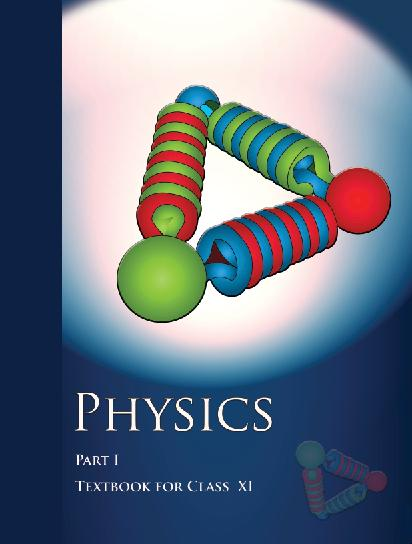## NCERT Class 11 Physics Chapter wise Solutions

• 1 – Physical World
• 2 – Units and Measurements
• 3 – Motion in a Straight line
• 4 – Motion in a Plane
• 5 – Laws of Motion
• 6 – Work, Energy and Power
• 7 – System of Particles and Rotational motion
• 8 – Gravitation
• 9 – Mechanical Properties of Solids
• 10 – Mechanical properties of fluids
• 11 – Thermal Properties of matter
• 12 – Thermodynamics
• 13 – Kinetic Theory
• 14 – Oscillations
• 15 – Waves

## CHAPTER 7 SYSTEM OF PARTICLES AND ROTATIONAL MOTION

• 7.1 Introduction
• 7.2 Centre of mass
• 7.3 Motion of centre of mass
• 7.4 Linear momentum of a system of particles
• 7.5 Vector product of two vectors
• 7.6 Angular velocity and its relation with linear velocity
• 7.7 Torque and angular momentum
• 7.8 Equilibrium of a rigid body
• 7.9 Moment of inertia
• 7.10 Theorems of perpendicular and parallel axes
• 7.11 Kinematics of rotational motion about a fixed axis
• 7.12 Dynamics of rotational motion about a fixed axis
• 7.13 Angular momentum in case of rotations about a fixed axis
• 7.14 Rolling motion

## NCERT Solutions For Class 11 Physics System of Particles and Rotational Motion Part 2

22. As shown in Fig.7.40, the two sides of a step ladder BA and CA are 1.6 m long and hinged at A. A rope DE, 0.5 m is tied half way up. A weight 40 kg is suspended from a point F, 1.2 m from B along the ladder BA. Assuming the floor to be frictionless and neglecting the weight of the ladder, find the tension in the rope and forces exerted by the floor on the ladder. (Take g = 9.8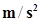)

(Hint: Consider the equilibrium of each side of the ladder separately.)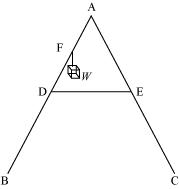23. A man stands on a rotating platform, with his arms stretched horizontally holding a 5 kg weight in each hand. The angular speed of the platform is 30 revolutions per minute. The man then brings his arms close to his body with the distance of each weight from the axis changing from 90cm to 20cm. The moment of inertia of the man together with the platform may be taken to be constant and equal to 7.6 kg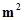.

(a) What is his new angular speed? (Neglect friction.)

(b) Is kinetic energy conserved in the process? If not, from where does the change come about?

24. A bullet of mass 10 g and speed 500 m/s is fired into a door and gets embedded exactly at the centre of the door. The door is 1.0 m wide and weighs 12 kg. It is hinged at one end and rotates about a vertical axis practically without friction. Find the angular speed of the door just after the bullet embeds into it.

(Hint: The moment of inertia of the door about the vertical axis at one end is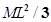.)

25. Two discs of moments of inertia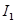and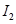about their respective axes (normal to the disc and passing through the centre), and rotating with angular speeds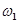and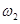are brought into contact face to face with their axes of rotation coincident.

(a) What is the angular speed of the two-disc system?

(b) Show that the kinetic energy of the combined system is less than the sum of the initial kinetic energies of the two discs. How do you account for this loss in energy? Take.

26. (a) Prove the theorem of perpendicular axes.

(Hint: Square of the distance of a point (x, y) in the x–y plane from an axis through the origin perpendicular to the plane is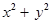).

(b) Prove the theorem of parallel axes.

(Hint: If the centre of mass is chosen to be the origin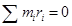).

27. Prove the result that the velocity v of translation of a rolling body (like a ring, disc, cylinder or sphere) at the bottom of an inclined plane of a height h is given by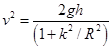.

Using dynamical consideration (i.e. by consideration of forces and torques). Note k is the radius of gyration of the body about its symmetry axis, and R is the radius of the body. The body starts from rest at the top of the plane.

28. A disc rotating about its axis with angular speed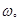is placed lightly (without any translational push) on a perfectly frictionless table. The radius of the disc is R. What are the linear velocities of the points A, B and C on the disc shown in Fig. 7.41? Will the disc roll in the direction indicated?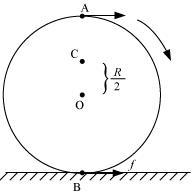29. Explain why friction is necessary to make the disc in Fig. 7.41 roll in the direction indicated.

(a) Give the direction of frictional force at B, and the sense of frictional torque, before perfect rolling begins.

(b) What is the force of friction after perfect rolling begins?

30. A solid disc and a ring, both of radius 10 cm are placed on a horizontal table simultaneously, with initial angular speed equal to 10 π rad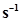. Which of the two will start to roll earlier? The co-efficient of kinetic friction is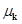= 0.2.

31. A cylinder of mass 10 kg and radius 15 cm is rolling perfectly on a plane of inclination 30°. The coefficient of static friction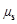= 0.25.

(a) How much is the force of friction acting on the cylinder?

(b) What is the work done against friction during rolling?

(c) If the inclination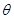of the plane is increased, at what value ofdoes the cylinder begin to skid, and not roll perfectly?

32. Read each statement below carefully, and state, with reasons, if it is true or false;

(a) During rolling, the force of friction acts in the same direction as the direction of motion of the CM of the body.

(b) The instantaneous speed of the point of contact during rolling is zero.

(c) The instantaneous acceleration of the point of contact during rolling is zero.

(d) For perfect rolling motion, work done against friction is zero.

(e) A wheel moving down a perfectly frictionless inclined plane will undergo slipping (not rolling) motion.

33. Separation of Motion of a system of particles into motion of the centre of mass and motion about the centre of mass:

(a) Show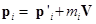Where pi is the momentum of the I th particle (of mass mi) and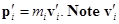is the velocity of the I th particle relative to the centre of mass.

Also, prove using the definition of the centre of mass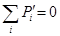(b) Show K =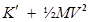Where K is the total kinetic energy of the system of particles,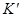is the total kinetic energy of the system when the particle velocities are taken with respect to the centre of mass and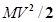is the kinetic energy of the translation of the system as a whole (i.e. of the centre of mass motion of the system). The result has been used in Sec.7.14.

(c) Show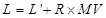Where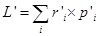is the angular momentum of the system about the centre of mass with velocities taken relative to the centre of mass . Remember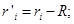rest of the notation is the standard notation used in the chapter. Note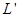and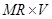can be said to be angular momenta, respectively, about and of the centre if mass of the system of particles.

(d) Show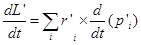Further show that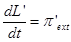Where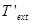is the sum of all external torques acting on the system about the centre of mass.

(Hint: Use the definition of centre of mass and Newton’s Third Law. Assume the internal forces between any two particles act along the line joining the particles.)

## NCERT Solutions for Class 11 Physics

NCERT Solutions Class 11 Physics PDF (Download) Free from myCBSEguide app and myCBSEguide website. Ncert solution class 11 physics includes text book solutions from both part 1 and part 2. NCERT Solutions for CBSE Class 11 Physics have total 15 chapters. 11 Physics NCERT Solutions in PDF for free Download on our website. Ncert physics class 11 solutions PDF and physics ncert class 11 PDF solutions with latest modifications and as per the latest CBSE syllabus are only available in myCBSEguide

## CBSE app for Class 11

To download NCERT Solutions for class 11 Physics, Chemistry, Biology, History, Political Science, Economics, Geography, Computer Science, Home Science, Accountancy, Business Studies and Home Science; do check myCBSEguide app or website. myCBSEguide provides sample papers with solution, test papers for chapter-wise practice, NCERT solutions, NCERT Exemplar solutions, quick revision notes for ready reference, CBSE guess papers and CBSE important question papers. Sample Paper all are made available through the best app for CBSE students and myCBSEguide website## Test Generator

Create Papers with your Name & Logo